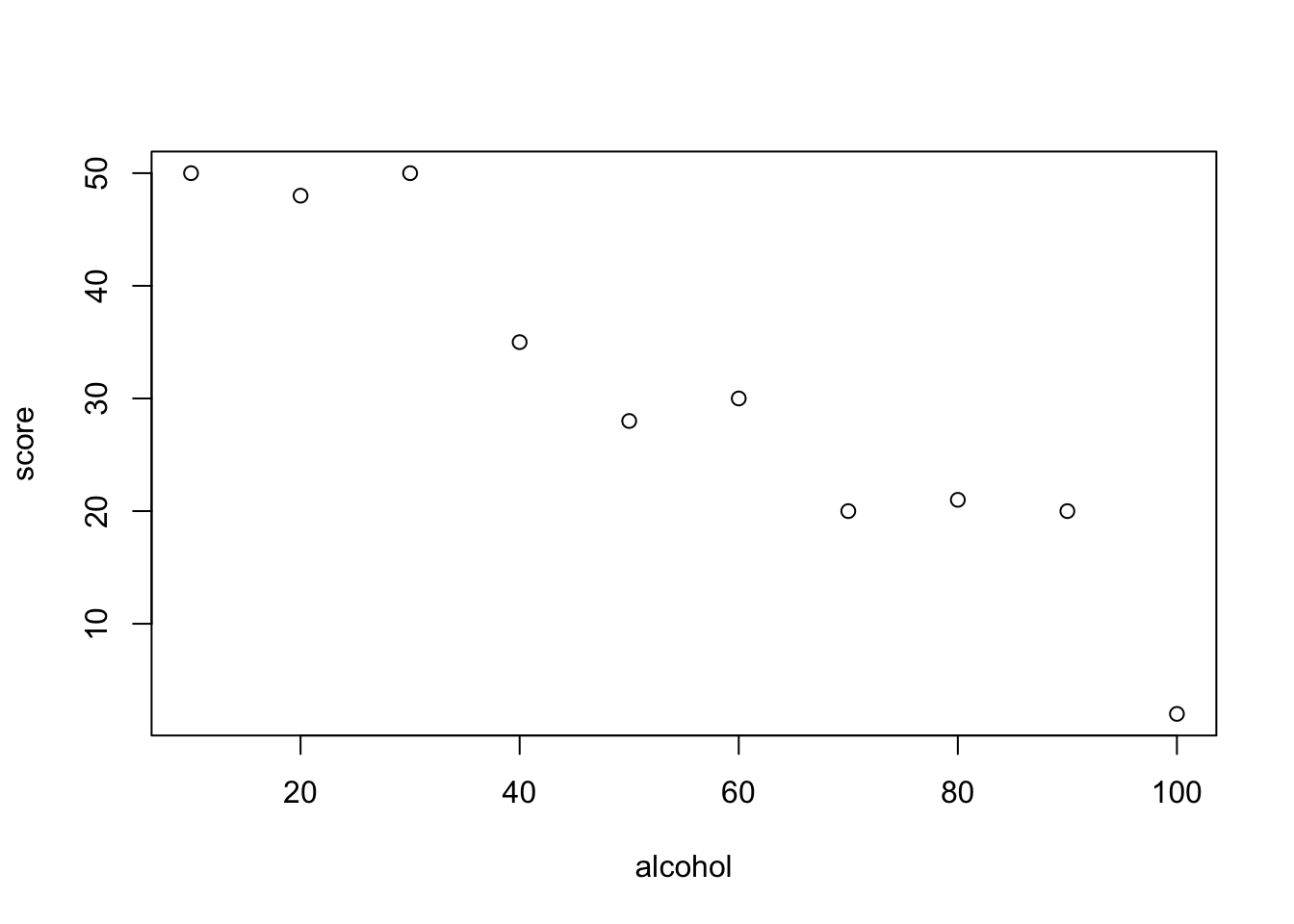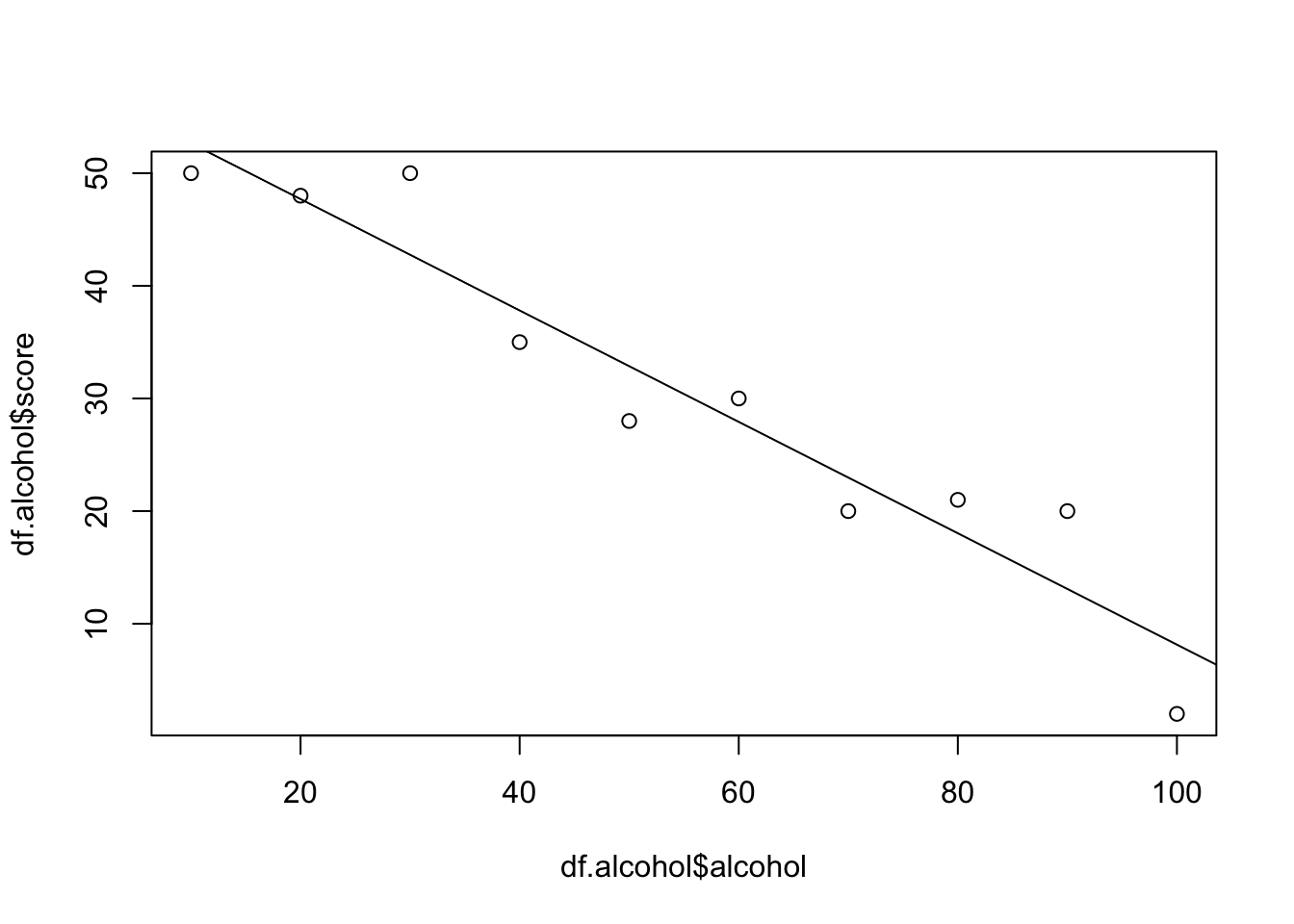# Introduction

The topic of this workshop is Bivariate regression - a very simple mathematical model, a line. We will go from the basics of bivariate regression in R to model inference and assumption checking. In the first hour we cover the basic with made up data and in the second hour we progress to the real life data.

This file is an R Markdown Notebook. When you execute code within the notebook, the results appear beneath the code. By using this format you can be introduced to the code with annotations and run sets of the code yourself. All of the code can run by pressing the play button above each cell, or clicking on options under the run button. You can also copy and paste the code into another file or the console.

# Data

We need some data. We will use fictional data.

## Our fictional study

Let us imagine we are interested on the influence of drinks consumed in our local drinking establishment. In particular, how does the amount of substances in drinks influence arcade game performance? We choose to do 3 studies looking at the influence of different amounts of 3 substances: artificial sweetner, alcohol and caffeine.

Our methodology for each substance is to:

1. Prepare 10 drinks with 10, 20, 30, 40, 50, 60, 70, 80, 90 and 100 of the substance mixed with water. (ml for alcohol and sweetner, mg for caffeine)
2. Choose 10 random people
3. For each person
1. After signing consent forms (keeping everything highly ethical!) give the person the drink to consume
2. Let them play an arcade game
3. Record their score

## Our data

Let us clear our R enviroment

rm(list=ls())

and create a data frame for each of our data sets. Each data frame is given fake data for model fitting purposes.

df.sweetner <- data.frame(sweetner = seq(10,100,10), score = c(50, 45, 55, 58, 55, 51, 59, 60, 50, 55))
df.alcohol <- data.frame(alcohol = seq(10,100,10), score = c(50, 48, 50, 35, 28, 30, 20, 21, 20, 2))
df.caffeine <- data.frame(caffeine = seq(10,100,10), score = c(42, 55, 60, 70, 68, 75, 78, 60, 50, 30))

We have a predictor (the substance amount) and an outcome (arcade game score) in each data set. These data appear perfect to fit a bivariate regression - a straight line model with one predictor and one outcome.

James will show you how to do this with the alcohol data set. Your task at the end of the demonstration is to try and fit a model to either the sweetner or caffeine data sets.

What does our data set look like? One way to see this is to print out the values.

df.alcohol

What do you notice about the impact of alcohol on score? Is there a relationship between the predictor and the outcome?

Perhaps a plot will make this clearer?

plot(df.alcohol)# A Regression!

We can describe the relationship between alcohol and score with a straight line! Technically this line is a linear model. The equation for this line is:

$score_i = \alpha + \beta \cdot alcohol_i + \epsilon$

This equation may look daunting but stick with it. The equation is to predict the value of score using an intercept (alpha), some random error (epsilon) and the corresponding value of alcohol multiplied by a value we call beta. It is up to R to find the straight line which gets as close to all the points as possible.

## Fitting the regression

We use a function called lm (linear model) to find the line which goes through the data.

m1 <- lm(formula = score~alcohol, data = df.alcohol)

An easy way to see this model is on a plot.

plot(df.alcohol$alcohol, df.alcohol$score)
abline(m1)R did a good job! The line goes through most of the data.

## A linear model?

But how does this relate to the equation you saw earlier? Has R figured out the value of the intercept (start of the line) and the coefficient for alcohol (value to multiply alcohol by)?

print(m1)
##
## Call:
## lm(formula = score ~ alcohol, data = df.alcohol)
##
## Coefficients:
## (Intercept)      alcohol
##     57.6000      -0.4945

Printing out m1 tells us the values R chose (the coefficients). The intercept is 57.6 and the coefficient for alcohol is -0.4945. Going back to the equation above, we can calculate a predicted score by multiplying a value of alcohol by -0.4945 and adding the intercept value.

Skeptical? I would be too. Let’s check and see if the values match up.

df.alcohol$predicted <- 57.6 + (df.alcohol$alcohol * -0.4945)
print(df.alcohol)
##    alcohol score predicted
## 1       10    50    52.655
## 2       20    48    47.710
## 3       30    50    42.765
## 4       40    35    37.820
## 5       50    28    32.875
## 6       60    30    27.930
## 7       70    20    22.985
## 8       80    21    18.040
## 9       90    20    13.095
## 10     100     2     8.150

That is pretty good. However, we still miss some points. For example, we miss the actual score when the dose of alcohol is 50ml by about 7. This error is the epsilon in the equation above. R found the straight line with the smallest errors. Well done R! We now know how to fit a bivariate regression model to data.

A small aside: we used a formula when fitting the linear model. Specifically, we told the lm function to create a linear model which predicts score using alccohol. Interestingly, this is a formula object. Note that we do not use apostrophes either side of the statement

class(score ~ alcohol)
##  "formula"

class('score ~ alcohol')
##  "character"

## Under the hood

What is this m1 variable? Does it contain only the coefficient values or is there more to it? Let us check the class

class(m1)
##  "lm"

and structure of m1

str(m1)
## List of 12
##  $coefficients : Named num [1:2] 57.6 -0.495 ## ..- attr(*, "names")= chr [1:2] "(Intercept)" "alcohol" ##$ residuals    : Named num [1:10] -2.655 0.291 7.236 -2.818 -4.873 ...
##   ..- attr(*, "names")= chr [1:10] "1" "2" "3" "4" ...
##  $effects : Named num [1:10] -96.13 -44.92 7.76 -2.21 -4.19 ... ## ..- attr(*, "names")= chr [1:10] "(Intercept)" "alcohol" "" "" ... ##$ rank         : int 2
##  $fitted.values: Named num [1:10] 52.7 47.7 42.8 37.8 32.9 ... ## ..- attr(*, "names")= chr [1:10] "1" "2" "3" "4" ... ##$ assign       : int [1:2] 0 1
##  $qr :List of 5 ## ..$ qr   : num [1:10, 1:2] -3.162 0.316 0.316 0.316 0.316 ...
##   .. ..- attr(*, "dimnames")=List of 2
##   .. .. ..$: chr [1:10] "1" "2" "3" "4" ... ## .. .. ..$ : chr [1:2] "(Intercept)" "alcohol"
##   .. ..- attr(*, "assign")= int [1:2] 0 1
##   ..$qraux: num [1:2] 1.32 1.27 ## ..$ pivot: int [1:2] 1 2
##   ..$tol : num 1e-07 ## ..$ rank : int 2
##   ..- attr(*, "class")= chr "qr"
##  $df.residual : int 8 ##$ xlevels      : Named list()
##  $call : language lm(formula = score ~ alcohol, data = df.alcohol) ##$ terms        :Classes 'terms', 'formula'  language score ~ alcohol
##   .. ..- attr(*, "variables")= language list(score, alcohol)
##   .. ..- attr(*, "factors")= int [1:2, 1] 0 1
##   .. .. ..- attr(*, "dimnames")=List of 2
##   .. .. .. ..$: chr [1:2] "score" "alcohol" ## .. .. .. ..$ : chr "alcohol"
##   .. ..- attr(*, "term.labels")= chr "alcohol"
##   .. ..- attr(*, "order")= int 1
##   .. ..- attr(*, "intercept")= int 1
##   .. ..- attr(*, "response")= int 1
##   .. ..- attr(*, ".Environment")=<environment: R_GlobalEnv>
##   .. ..- attr(*, "predvars")= language list(score, alcohol)
##   .. ..- attr(*, "dataClasses")= Named chr [1:2] "numeric" "numeric"
##   .. .. ..- attr(*, "names")= chr [1:2] "score" "alcohol"
##  $model :'data.frame': 10 obs. of 2 variables: ## ..$ score  : num [1:10] 50 48 50 35 28 30 20 21 20 2
##   ..$alcohol: num [1:10] 10 20 30 40 50 60 70 80 90 100 ## ..- attr(*, "terms")=Classes 'terms', 'formula' language score ~ alcohol ## .. .. ..- attr(*, "variables")= language list(score, alcohol) ## .. .. ..- attr(*, "factors")= int [1:2, 1] 0 1 ## .. .. .. ..- attr(*, "dimnames")=List of 2 ## .. .. .. .. ..$ : chr [1:2] "score" "alcohol"
##   .. .. .. .. ..$: chr "alcohol" ## .. .. ..- attr(*, "term.labels")= chr "alcohol" ## .. .. ..- attr(*, "order")= int 1 ## .. .. ..- attr(*, "intercept")= int 1 ## .. .. ..- attr(*, "response")= int 1 ## .. .. ..- attr(*, ".Environment")=<environment: R_GlobalEnv> ## .. .. ..- attr(*, "predvars")= language list(score, alcohol) ## .. .. ..- attr(*, "dataClasses")= Named chr [1:2] "numeric" "numeric" ## .. .. .. ..- attr(*, "names")= chr [1:2] "score" "alcohol" ## - attr(*, "class")= chr "lm" It seems there is a lot more in the m1 variable. Where should we start? Well, there are the coefficients m1$coefficients
## (Intercept)     alcohol
##  57.6000000  -0.4945455

and also the errors

m1$residuals ## 1 2 3 4 5 6 ## -2.6545455 0.2909091 7.2363636 -2.8181818 -4.8727273 2.0727273 ## 7 8 9 10 ## -2.9818182 2.9636364 6.9090909 -6.1454545 which are the difference between the actual score and the predicted score df.alcohol$score - df.alcohol$predicted ##  -2.655 0.290 7.235 -2.820 -4.875 2.070 -2.985 2.960 6.905 -6.150 and the original data m1$model

There is more to explore but, alas, we must press on.

We can do more things with a model object (variable) by passing it to a function. A common function used with linear models is summary. The summary function produced details about inferential statistics.

summary(m1)
##
## Call:
## lm(formula = score ~ alcohol, data = df.alcohol)
##
## Residuals:
##    Min     1Q Median     3Q    Max
## -6.146 -2.941 -1.182  2.741  7.236
##
## Coefficients:
##             Estimate Std. Error t value Pr(>|t|)
## (Intercept) 57.60000    3.40414  16.921 1.51e-07 ***
## alcohol     -0.49455    0.05486  -9.014 1.83e-05 ***
## ---
## Signif. codes:  0 '***' 0.001 '**' 0.01 '*' 0.05 '.' 0.1 ' ' 1
##
## Residual standard error: 4.983 on 8 degrees of freedom
## Multiple R-squared:  0.9104, Adjusted R-squared:  0.8992
## F-statistic: 81.26 on 1 and 8 DF,  p-value: 1.832e-05

We will go into more detail about this output in the next file. Suffice to say, many people fit linear models explicitely to get this output and in our case indicates alcohol influences score.

## Making predictions

Another function used with linear models is predict. We can ask R what the model predicts for new data. Imagine we gave people even more alcohol.

df.alcohol.more <- data.frame(alcohol = c(200, 250, 300))

We ask R to predict the score using the linear model already fit. In other words, take each new alcohol value and work out the predicted score.

$score_i = 57.6 + (-0.49455 \cdot alcohol_i)$

We do this using the predict function

predict(m1, df.alcohol.more)
##         1         2         3
## -41.30909 -66.03636 -90.76364

We can this add new data alonside the old.

plot(c(0,300), c(-100, 100), type='n', xlab = 'Alcohol (ml)', ylab = 'Score') # make blank plot
points(df.alcohol$alcohol, df.alcohol$score, col="blue", pch = 15)
points(df.alcohol.more\$alcohol, predict(m1, df.alcohol.more), col="red", pch = 8)
abline(m1)
legend('bottomleft',
legend = c('Data', 'Predicted Data', 'Model'),
col = c('blue', 'red', 'black'),
pch = c(15, 8, 9),
text.col = 'Black')